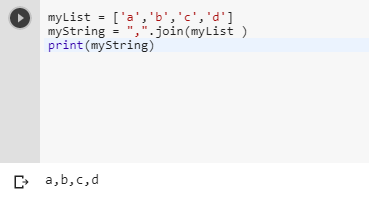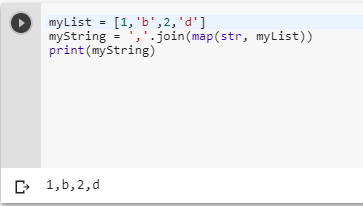2 views
in Python

What would be your preferred way to concatenate strings from a sequence such that between each two consecutive pair a comma is added. That is, how do you map, for instance, [ 'a', 'b', 'c' ]to 'a,b,c'? (The case [ s ] and [] should be mapped to s and '', respectively.)

I usually end up using something like ''.join(map(lambda x: x+',',l))[:-1], but also feeling somewhat unsatisfied.

Edit: I'm both ashamed and happy that the solution is so simple. Obviously, I have hardly a clue as to what I'm doing. (I probably needed "simple" concatenation in the past and somehow memorised s.join([e1,e2,...]) as a shorthand for s+e1+e2+....)

by (106k points)

# To make a comma-separated string from a list of strings you can use join() function:-

myList = ['a','b','c','d']

myString = ",".join(myList )

print(myString)But the above function will not work if the list contains numbers. So in the case if list containing numbers you can use map with join() function.

myList = [1,'b',2,'d']

myString = ','.join(map(str, myList))

print(myString)